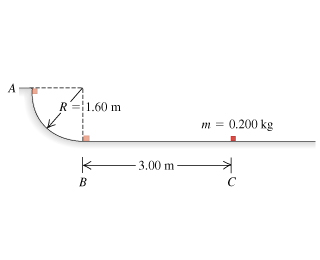# Problem: In a truck-loading station at a post office, a small 0.200-kg package is released from rest at point exttip{A}{A} on a track that is one-quarter of a circle with radius 1.60 m (the figure ). The size of the package is much less than 1.60 m, so the package can be treated as a particle. It slides down the track and reaches point exttip{B}{B} with a speed of 4.60 m/s . From point exttip{B}{B}, it slides on a level surface a distance of 3.00 m to point C, where it comes to rest.What is the coefficient of kinetic friction on the horizontal surface?How much work is done on the package by friction as it slides down the circular arc from exttip{A}{A} to exttip{B}{B}?

⚠️Our tutors found the solution shown to be helpful for the problem you're searching for. We don't have the exact solution yet.

###### Problem Details

In a truck-loading station at a post office, a small 0.200-kg package is released from rest at point on a track that is one-quarter of a circle with radius 1.60 m (the figure). The size of the package is much less than 1.60 m, so the package can be treated as a particle. It slides down the track and reaches point with a speed of 4.60 m/s . From point , it slides on a level surface a distance of 3.00 m to point C, where it comes to rest.

What is the coefficient of kinetic friction on the horizontal surface?

How much work is done on the package by friction as it slides down the circular arc from to ?